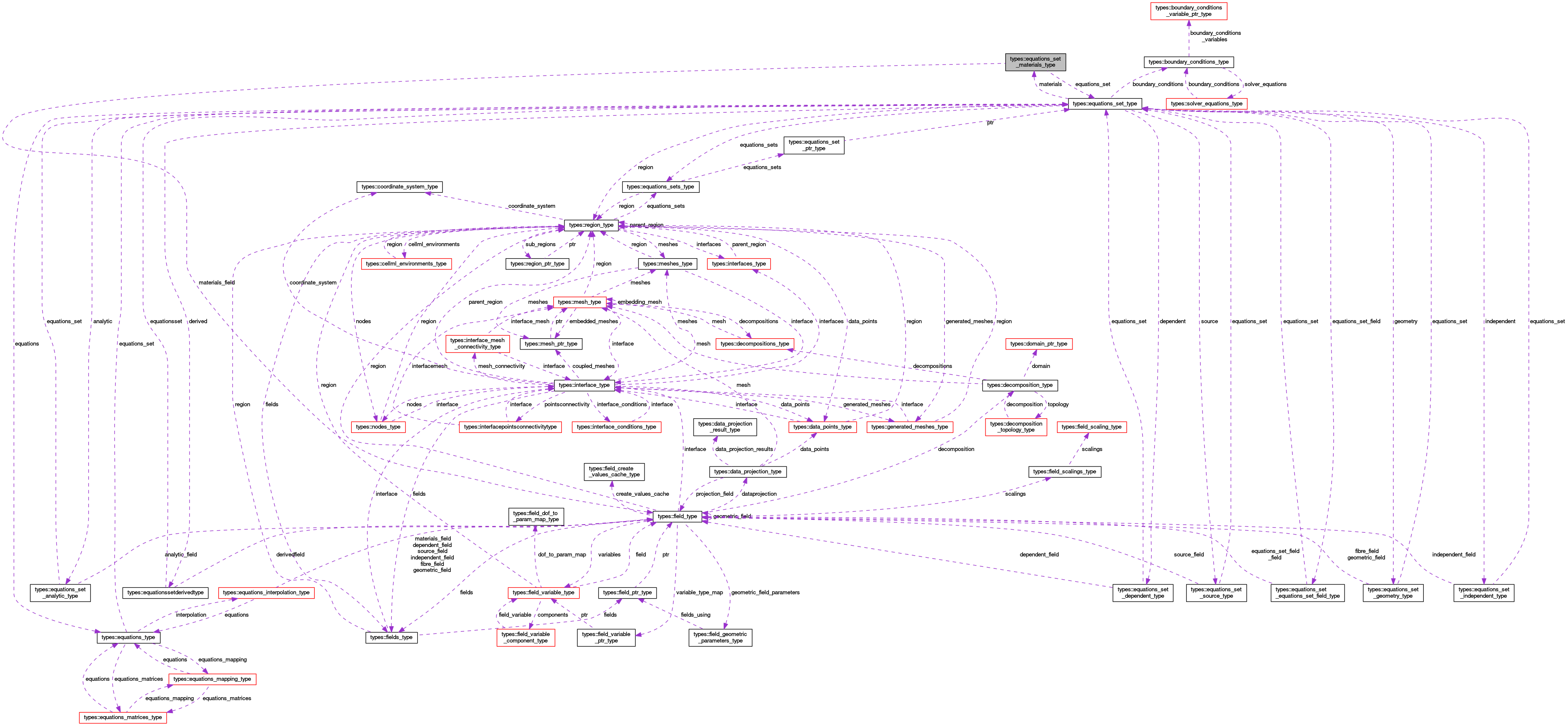OpenCMISS-Iron Internal API Documentation
types::equations_set_materials_type Type Reference
Collaboration diagram for types::equations_set_materials_type:[legend]

## Public Attributes

type(equations_set_type), pointer equations_set
A pointer to the equations set. More...

logical materials_finished
Is .TRUE. if the materials for the equations set has finished being created, .FALSE. if not. More...

logical materials_field_auto_created
Is .TRUE. if the materials field has been auto created, .FALSE. if not. More...

type(field_type), pointer materials_field
A pointer to the materials field for the equations set if one is defined. If no material field is defined the pointer is NULL. More...

## Detailed Description

Definition at line 1881 of file types.f90.

## Member Data Documentation

 type(equations_set_type), pointer types::equations_set_materials_type::equations_set

A pointer to the equations set.

Definition at line 1882 of file types.f90.

 type(field_type), pointer types::equations_set_materials_type::materials_field

A pointer to the materials field for the equations set if one is defined. If no material field is defined the pointer is NULL.

Definition at line 1885 of file types.f90.

 logical types::equations_set_materials_type::materials_field_auto_created

Is .TRUE. if the materials field has been auto created, .FALSE. if not.

Definition at line 1884 of file types.f90.

 logical types::equations_set_materials_type::materials_finished

Is .TRUE. if the materials for the equations set has finished being created, .FALSE. if not.

Definition at line 1883 of file types.f90.